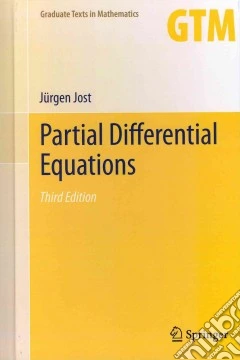ricerca
avanzata

# Partial Differential Equations

## Un libro in lingua di Jurgen Jost edito da Springer Verlag, 2012

• € 81,00
• Il prezzo è variabile in funzione del cambio della valuta d’origineThis book offers an ideal graduate-level introduction to the theory of partial differential equations. The first part of the book describes the basic mathematical problems and structures associated with elliptic, parabolic, and hyperbolic partial differential equations, and explores the connections between these fundamental types. Aspects of Brownian motion or pattern formation processes are also presented. The second part focuses on existence schemes and develops estimates for solutions of elliptic equations, such as Sobolev space theory, weak and strong solutions, Schauder estimates, and Moser iteration. In particular, the reader will learn the basic techniques underlying current research in elliptic partial differential equations.This revised and expanded third edition is enhanced with many additional examples that will help motivate the reader. New features include a reorganized and extended chapter on hyperbolic equations, as well as a new chapter on the relations between different types of partial differential equations, including first-order hyperbolic systems, Langevin and Fokker-Planck equations, viscosity solutions for elliptic PDEs, and much more. Also, the new edition contains additional material on systems of elliptic partial differential equations, and it explains in more detail how the Harnack inequality can be used for the regularity of solutions.